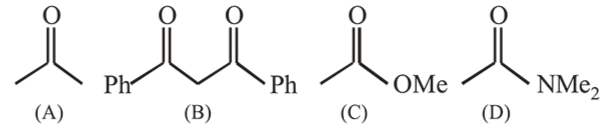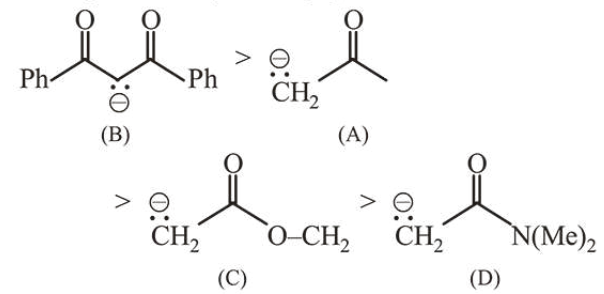# The increasing order of the acidity of the

Question:

The increasing order of the acidity of the $\alpha$-hydrogen of the following compounds is :1. $(\mathrm{D})<(\mathrm{C})<(\mathrm{A})<(\mathrm{B})$

2. $(B)<(C)<(A)<(D)$

3. $(\mathrm{A})<(\mathrm{C})<(\mathrm{D})<(\mathrm{B})$

4. $(C)<(A)<(B)<(D)$

Correct Option: 1

Solution:

(a) Acidity $\propto$ stability of conjugate baseThus increasing order of acidity is $\mathrm{D}<\mathrm{C}<\mathrm{A}<\mathrm{B}$.